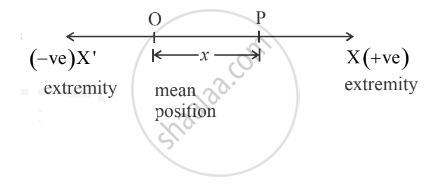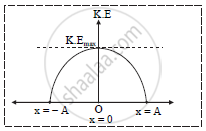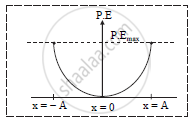Share

# Prove the Law of Conservation of Energy for a Particle Performing Simple Harmonic Motion. Hence Graphically Show the Variation of Kinetic Energy and Potential Energy W. R. T. - Physics

#### Question

Prove the law of conservation of energy for a particle performing simple harmonic motion.Hence graphically show the variation of kinetic energy and potential energy w. r. t. instantaneous displacement.

#### Solution

i. Suppose a particle of mass m performing linear S.H.M. is at point P which is at a distance x
from the mean position O as shown in figure.ii. Kinetic energy of particle at point P is given by,

K.E=1/2momega^2(A^2-x^2)

iii. Potential energy at point P is given by,

P.E=1/2momega^2x^2

iv.   Total energy at point P is given by,
T.E. = K.E. + P.E

=1/2 momega^2(A^2-x^2)+1/2momega^2x^2

=1/2momega^2(A^2-x^2+x^2)

T.E=1/2momega^2A^2............(1)

v.   If particle is at mean position:
x = 0

therefore K.E=1/2 momega^2A^2

P.E=1/2momega^2xx0=0

therefore T.E=K.E+P.E=1/2momega^2A^2...............(2)

vi.   If particle is at extreme position:
x = A

K.E=1/2momega^2(A^2-A^2)=0

P.E=1/2 momega^2A^2

therefore T.E=P.E+K.E=1/2 momega^2A^2...............(3)

vii. From equations (1), (2) and (3), it is observed that total energy of a particle performing linear S.H.M. at any point in its path is constant. Hence, total energy of linear S.H.M.  remains conserved.

viii. a. Graph of variation of kinetic energy w. r. t. instantaneous displacement.b. Graph of variation of potential energy w.r.t. instantaneous displacementIs there an error in this question or solution?